Test: Time Response

# Test: Time Response - Electrical Engineering (EE)

Test Description

## 20 Questions MCQ Test GATE Electrical Engineering (EE) 2024 Mock Test Series - Test: Time Response

Test: Time Response for Electrical Engineering (EE) 2023 is part of GATE Electrical Engineering (EE) 2024 Mock Test Series preparation. The Test: Time Response questions and answers have been prepared according to the Electrical Engineering (EE) exam syllabus.The Test: Time Response MCQs are made for Electrical Engineering (EE) 2023 Exam. Find important definitions, questions, notes, meanings, examples, exercises, MCQs and online tests for Test: Time Response below.
Solutions of Test: Time Response questions in English are available as part of our GATE Electrical Engineering (EE) 2024 Mock Test Series for Electrical Engineering (EE) & Test: Time Response solutions in Hindi for GATE Electrical Engineering (EE) 2024 Mock Test Series course. Download more important topics, notes, lectures and mock test series for Electrical Engineering (EE) Exam by signing up for free. Attempt Test: Time Response | 20 questions in 60 minutes | Mock test for Electrical Engineering (EE) preparation | Free important questions MCQ to study GATE Electrical Engineering (EE) 2024 Mock Test Series for Electrical Engineering (EE) Exam | Download free PDF with solutions
 1 Crore+ students have signed up on EduRev. Have you?
Test: Time Response - Question 1

### The open-loop transfer function of a ufb control system is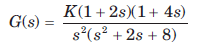The position, velocity and acceleration error constants are respectively

Detailed Solution for Test: Time Response - Question 1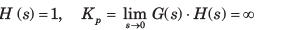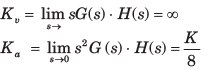Test: Time Response - Question 2

### The open-loop transfer function of a unit feedback system is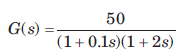The position, velocity and acceleration errorconstants are respectively

Detailed Solution for Test: Time Response - Question 2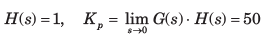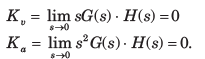Test: Time Response - Question 3

### If a type 0 system is subjected to step input, what is its effect on steady state error?

Test: Time Response - Question 4

The forward-path transfer function of a unity feedback system is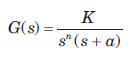The system has 10% overshoot and velocity error constant Kv =100.

The value of a is

Detailed Solution for Test: Time Response - Question 4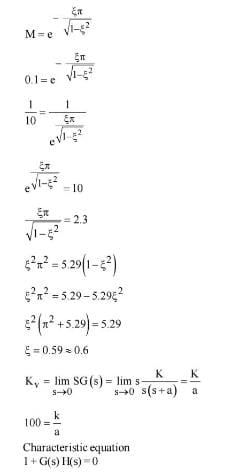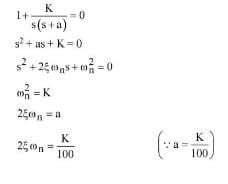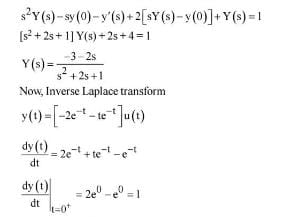Test: Time Response - Question 5

For the system shown in fig.the steady state error component due to unit step disturbance is 0.000012 and steady state error component due to unit ramp input is 0.003. The values of K1 and K2 are respectively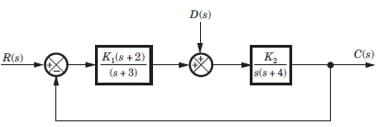Detailed Solution for Test: Time Response - Question 5

If R (s) = 0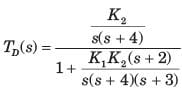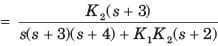Error in output due to disturbance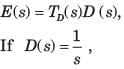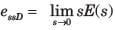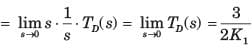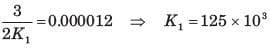Error due to ramp input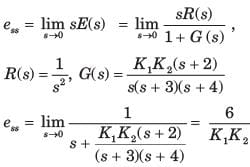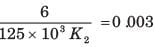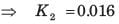Test: Time Response - Question 6

The transfer function for a single loop nonunity feedback control system is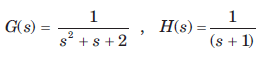The steady state error due to unit step input is

Detailed Solution for Test: Time Response - Question 6

E(s) = R(s) - C(s) H(s)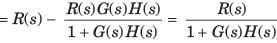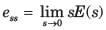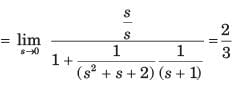Test: Time Response - Question 7

For the system of fig. the total steady state error due to a unit step input and a unit step disturbance is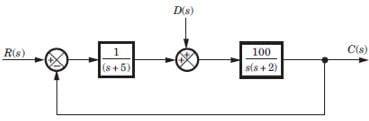Detailed Solution for Test: Time Response - Question 7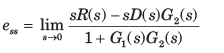where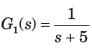and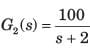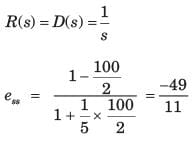Test: Time Response - Question 8

The forward path transfer function of a ufb system is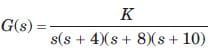If a unit ramp is applied, the minimum possiblesteady-state error is

Detailed Solution for Test: Time Response - Question 8

Using Routh-Hurwitz Criterion, system is stable for 0 < K < 2000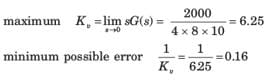Test: Time Response - Question 9

The forward-path transfer function of a ufb system is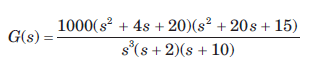The system has r(t) = t3 applied to its input. Thesteady state error is

Detailed Solution for Test: Time Response - Question 9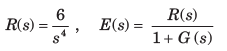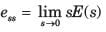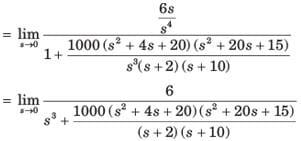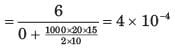Test: Time Response - Question 10

Consider a unity feedback system with forward transfer function given by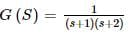The steady-state error in the output of the system for a unit-step input is _________ (up to 2 decimal places).

Detailed Solution for Test: Time Response - Question 10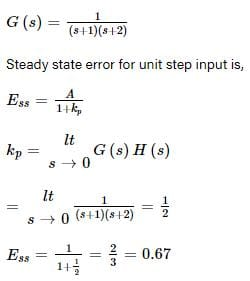Test: Time Response - Question 11

A system has position error constant Kp  = 3. The steady state error for input of 8tu(t) is

Detailed Solution for Test: Time Response - Question 11

System is zero type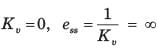Test: Time Response - Question 12

The forward path transfer function of a unity feedback system is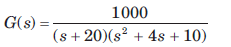For input of 60u(t) steady state error is

Detailed Solution for Test: Time Response - Question 12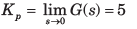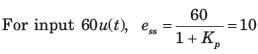Test: Time Response - Question 13

For ufb system shown in fig. the transfer function is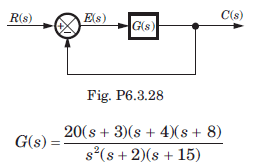If input is 30t2 , then steady state error is

Detailed Solution for Test: Time Response - Question 13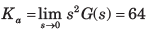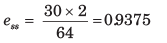Test: Time Response - Question 14

The forward-path transfer function of a ufb control system is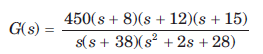The steady state errors for the test input 37tu(t) is

Detailed Solution for Test: Time Response - Question 14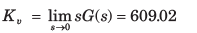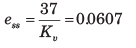Test: Time Response - Question 15

In the system shown in fig. r(t) =  1 + 2t , t > 0. The steady state error e(t) is equal to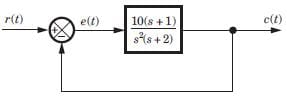Detailed Solution for Test: Time Response - Question 15

The system is type 2. Thus to step and ramp input error will be zero.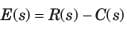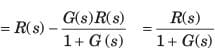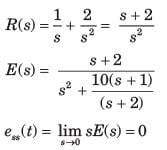Test: Time Response - Question 16

A ufb control system has a forward path transfer function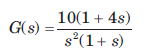If the system is subjected to an input r(t) = 1 + t + 1/2 t2 , t > 0the steady state error of the system will be

Detailed Solution for Test: Time Response - Question 16

Explanation : R(s) = (1/s)+(1/s2) + (1/s3)

E(s) = R(s) / {1 + G(s)} = 1/10s

ess = lim sE(s) = 0.1

Test: Time Response - Question 17

The system shown in fig. has steady-state error 0.1 to unit step input. The value of K is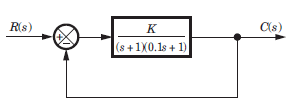Detailed Solution for Test: Time Response - Question 17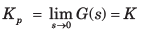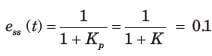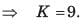Test: Time Response - Question 18

Block diagram of a position control system is shown in fig.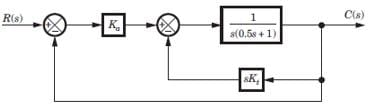If Kt = 0 and Ka= 5, then the steady state error tounit ramp input is

Detailed Solution for Test: Time Response - Question 18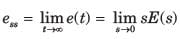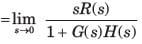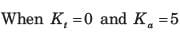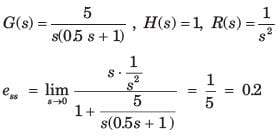Test: Time Response - Question 19

Block diagram of a position control system is shown in fig.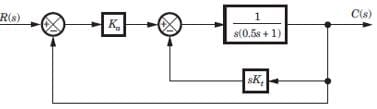If the damping ratio of the system is increased to 0.7 without affecting the steady state error, then thevalue of Ka and Kt are

Detailed Solution for Test: Time Response - Question 19

The equivalent open-loop transfer function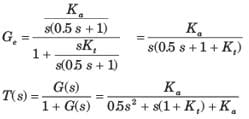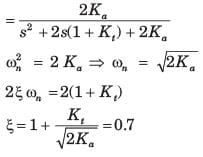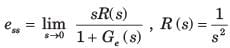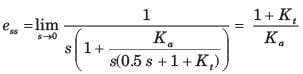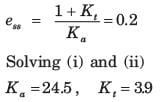Test: Time Response - Question 20

A system has the following transfer function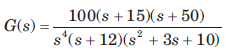The type and order of the system are respectively

Detailed Solution for Test: Time Response - Question 20

The s has power of 4 and denominator has order of 7. So Type 4 and Order 7.

## GATE Electrical Engineering (EE) 2024 Mock Test Series

23 docs|285 tests
Information about Test: Time Response Page
In this test you can find the Exam questions for Test: Time Response solved & explained in the simplest way possible. Besides giving Questions and answers for Test: Time Response, EduRev gives you an ample number of Online tests for practice

## GATE Electrical Engineering (EE) 2024 Mock Test Series

23 docs|285 tests